# Begining Circuit Analysis and Ohms LawCircuit analysis is the process of looking at a circuit and figuring out what it does. For simple circuits this is pretty easy. For complex circuits it can be amazingly hard, even for pros. This guide will get you started with circuit analysis.

Lets start with a simple circuit and figure out what it does. If you have been reading these guides from the begining you have seen this circuit before.

 Schematic Symbol Actual Image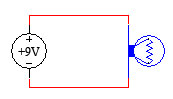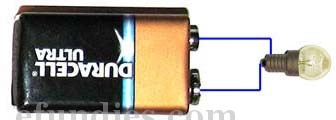Lets analyze this circuit.

• The battery provides +9 volts and ground to the light bulb through wires.
• The light bulb uses the voltage provided to create light.
• Over time, the battery will drain and the light bulb will no longer light up.

This is a good analysis of the circuit above. It seems that the battery has energy stored up in it, and the light bulb is using that energy to create light. Over time the energy is depleted and the battery is dead.

Batteries and Voltage

Batteries are energy storing devices. They are frequently rated in volts because a certain voltage is present at the + (positive) terminal of a battery.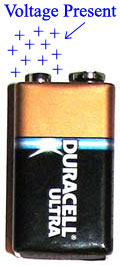This battery has voltage present on its + terminal.

Voltage is a measurement of potential energy. This means that voltage has the potential to do work. It does not do work on its own, it just has the potential to do it. Voltage is a measurement, just like inches or centimenters is a measurement. Inches and centimeters measure length, while voltage measures potential energy.

When voltage (potential energy) is present in a closed circuit, it causes a current to flow. Current is the flow of electricity from one location to another. Current is measured in amps. Lets look at a table of different measurements to clarify.

 What is being measured How it is measured Distance Inches Distance Centimeters Voltage Potential Volts Current Amps

See that Voltage Potential is measured in Volts while Current is measured in Amps. You’ll want to remember that for later.

Current Flow

Now lets look at how current flows in a circuit.

 Schematic Symbol Actual Image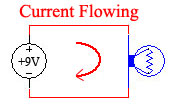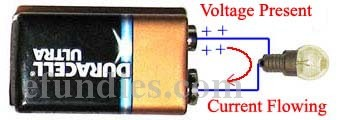Current flows from the + (positive) terminal of a battery, through whatever is connected to it, and to the – (negative) terminal of the battery. Remember that we frequently call the negative terminal of the battery ground.

It is not possible to have current unless you have a closed circuit. As soon as the circuit is opened, the current stops flowing. However, in an open circuit the voltage remains present.

 Schematic Symbol Actual Image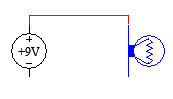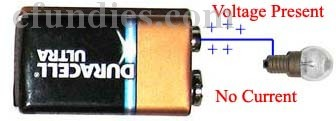In the picture above the circuit is open so there is no current flowing. However voltage is still present in the circuit.

The Voltage to Water Analogy

A common way to think about voltage and current is to think about water in a lake. Voltage is like the amount of water present, whether it is moving or not. Current is the amount of water flowing.The lake is a like battery with a large voltage. The river flowing out is current.

When you have a large body of water, you have the potential of having a large current. Similarly, when you have a large voltage you have the potential of having a large current.

Resistance

There is one more thing that we need to talk about when we are talking about voltage and current. We need to talk about Resistance. Generally the term resistance is applied to all electrical components that are not batteries. With the circuit we’ve been using in this guide there is only one component that is not a battery. The light bulb in this circuit is the only component that is not a battery.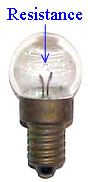A light bulb has a set amount of resistance.

All light bulbs have a set amount of resistance that is built in to them. This resistance does not change through out the bulbs life (until it stops working, when it becomes an open circuit). The amount of resistance in the bulb is directly related to how bright it gets. Resistance is measured in Ohms.

 What is being measured How it is measured Distance Inches Distance Centimeters Voltage Potential Volts Current Amps Resistance Ohms

You can see from the table above that Resistance is measured in Ohms.

Back to The Water Analogy

Looking back at the water analogy, the amount of water in the lake is the same as voltage in a battery, while the amount of water flowing out of the lake is the same as the current in a circuit. Now we can add that the size of the opening in the dam for water to come out is the same as the resistance in a circuit. In other words:

Resistors limit the current, or flow, in a circuit.

Resistors resist, or restric the flow of electricity in a circuit. Now that we understand Voltage, Current and Resistance, we are ready to learn Ohms Law which relates all three of them together.

Ohms Law

We know that current is flowing in a circuit. It would be handy sometimes to be able to figure out how much current is flowing. It turns out there is an equation that links voltage to current and resistance. That equation is called Ohms Law.

Ohms Law will seem difficult to grasp at first. Do not be disappointed if you do not understand it completly. The purpose of this guide is to introduce you to Ohms Law so that you can understand why it is important. As you progress in your knowledge of electronics, you should return to this guide a few times to remind yourself of what Ohms Law means.

The Law

Here is Ohms Law, in its most common form.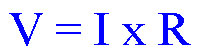Ohms Law: V = I x R

In Ohms Law, V stands for Voltage and R stands for Resistance. Those 2 are easy. The hard one to remember is that I stands for Current. This equation tells us that Voltage equals Current times Resistance. If we know current and resistance, we can calculate voltage.

Using algegra, we can express Ohms Law in 2 other forms. Lets look at those forms now.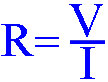Ohms Law, form 2: R = V / I

In this form, Ohms law is telling us that Resistance is equal to Voltage divided by Current. In this form if we know voltage and current we can calculate resistance.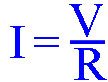Ohms Law, form 3: I = V / R

Finally, this equation tells us that Current equals Voltage divided by Resistance.

If you are familiar with algebra then you may notice that all 3 forms are really the same equation. If you are not familiar with algebra then you will just have to take my word for it. You can use any of the above 3 equations any time you need to use Ohms Law. They are interchangeable.

Using Ohms Law

Lets use Ohms Law to figure out how much current is flowing through a circuit.

 Schematic Symbol Actual ImageWe want to figure out the current flowing through the light bulb, so lets use form 3 of Ohms Law:Ohms Law, form 3: I = V / R

In this case, I is the current flowing through the light bulb, V is the voltage of the battery, and R is the resistance of the light bulb.

We can fill in V because we know that it equals +9 volts.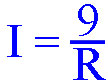Current equals 9 divided by R.

If we only knew the Resistance of the light bulb, then we could calculate how much current is flowing through it. It turns out that finding the resistance of a device is pretty easy, if you have a meter to do it with. For now, I’ll just tell you that the light bulb we are using here has 2 ohms of resistance. I used my ohmmeter to determine the resistance of the bulb, which we talk about in our How to Use a Multimeter guide.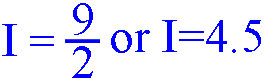Referring back to the table that says Current is measured in Amps, our answer is I = 4.5 Amps. Using Ohms law we have calculated that 4.5 Amps of current are flowing through a 2 ohm light bulb when hooked up to a 9 volt battery. By knowing the voltage of the battery and measuring the resistance of the light bulb, we are able to calculate the current flowing through it.

Ohms Law is used throughout electronics to figure out how a circuit works and why it is either functioning or not functioning. It is very important that you understand everything discussed in this guide. If you are still fuzzy at this point, then take a break and come back to it again later.

This site uses Akismet to reduce spam. Learn how your comment data is processed.